2-2 Iris Dataset

[chinese][all]

Iris dataset is by far the earliest and the most commonly used dataset in the literature of pattern recognition. The dataset contains 150 instances of iris flowers collected in Hawaii. These instances are divided into 3 classes of Iris Setosa, Iris Versicolour and Iris Virginica, based on 4 measures of sepal's width and length, and petal's width and length. These measures are taken for each iris flower, as shown next: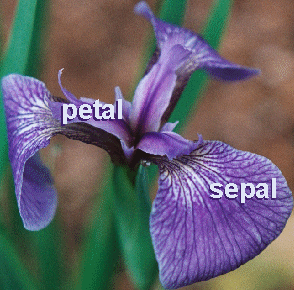Typical examples of these 3 iris species are shown next:Iris Setosa (More info) Iris Versicolor (More info) Iris Virginica (More info)
Detailed information of the dataset is listed next:
• 4 features with numerical values, with no missing data
• sepal length in cm
• sepal width in cm
• petal length in cm
• petal width in cm
• 3 classes, including Iris Setosa, Iris Versicolour, Iris Virginica
• data size: 150 entries
• data distribution: 50 entries for each class
There are numerous technical papers that use Iris dataset. Here is a partial list:
1. Fisher,R.A. "The use of multiple measurements in taxonomic problems" Annual Eugenics, 7, Part II, 179-188 (1936); also in "Contributions to Mathematical Statistics" (John Wiley, NY, 1950).
2. Duda,R.O., & Hart,P.E. (1973) Pattern Classification and Scene Analysis. (Q327.D83) John Wiley & Sons. ISBN 0-471-22361-1. See page 218.
3. Dasarathy, B.V. (1980) "Nosing Around the Neighborhood: A New System Structure and Classification Rule for Recognition in Partially Exposed Environments". IEEE Transactions on Pattern Analysis and Machine Intelligence, Vol. PAMI-2, No. 1, 67-71.
4. Gates, G.W. (1972) "The Reduced Nearest Neighbor Rule". IEEE Transactions on Information Theory, May 1972, 431-433.

In the dataset, Iris Setosa is easier to be distinguished from the other two classes, while the other two classes are partially overlapped and harder to be separated.

We can display the data sizes among all classes, as follows:

Example 1: irisClassDataCount01.mds=prData('iris'); [classSize, classLabel]=dsClassSize(ds, 1); 4 features 150 instances 3 classes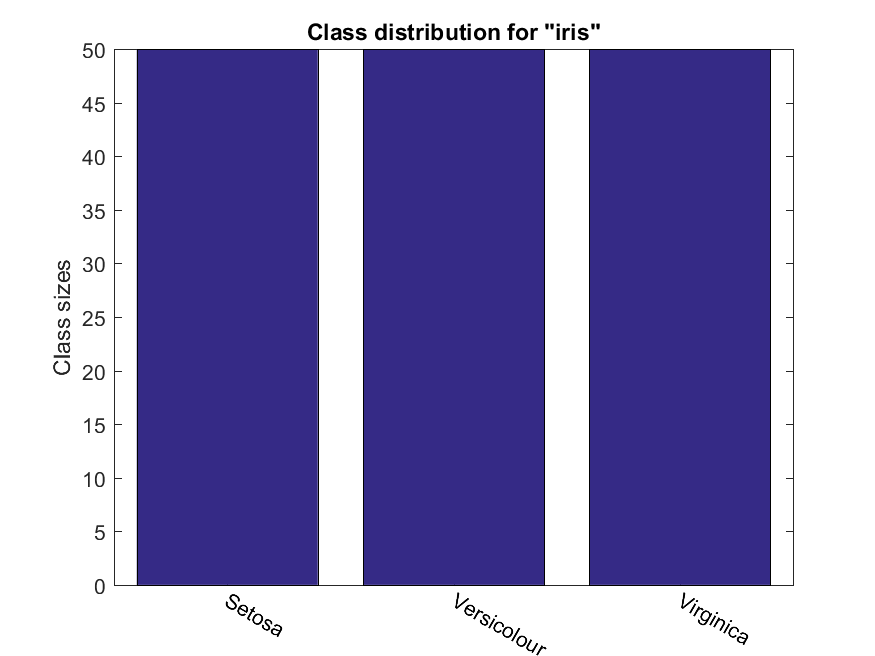We can display the feature distributions over different classes, as follows:

Example 2: irisClassDist01.mds=prData('iris'); dsDistPlot(ds);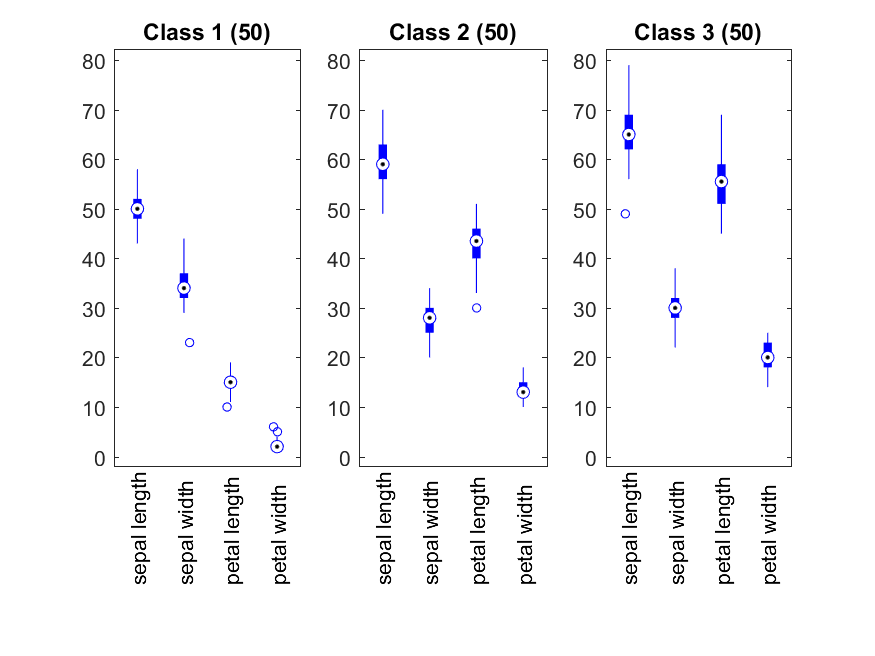We can plot the classes w.r.t. each of the features:

Example 3: irisProjPlot1.mds = prData('iris'); dsProjPlot1(ds);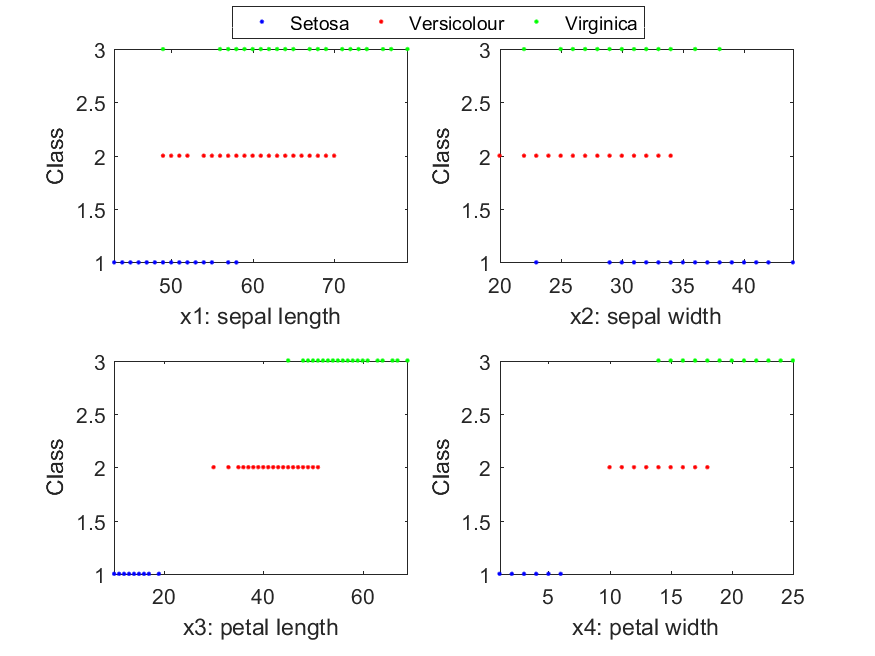We can have a scatter plot after projecting the dataset onto a 2D plane:

Example 5: irisProjPlot2.mds = prData('iris'); dsProjPlot2(ds);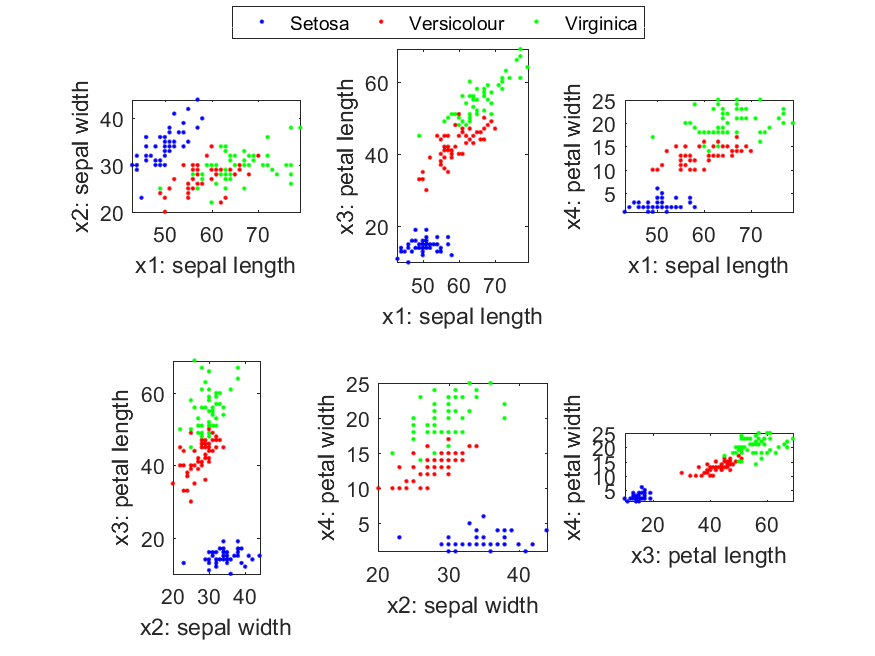From the above plot, it seems that the projection over features 3 and 4 can separate the classes most appropriately. If we need to identify a point in the scatter plot, we need to put annotation to each data point first. Then when we draw the mouse near a specific data point, the corresponding annotation will appear. The next example demonstrate how to achieve this. Please move the cursor to any point to see the corresponding annotation.

Example 6: irisPlot2dWithAnnotation.mds=prData('iris'); ds.input=ds.input(3:4, :); for i=1:length(ds.output) ds.annotation{i}=sprintf('Data index=%d\nPosition=%s\nClass=%s', i, mat2str(ds.input(:,i)), ds.outputName{ds.output(i)}); end opt.showAnnotation=1; opt.showLegend=1; dsScatterPlot(ds, opt);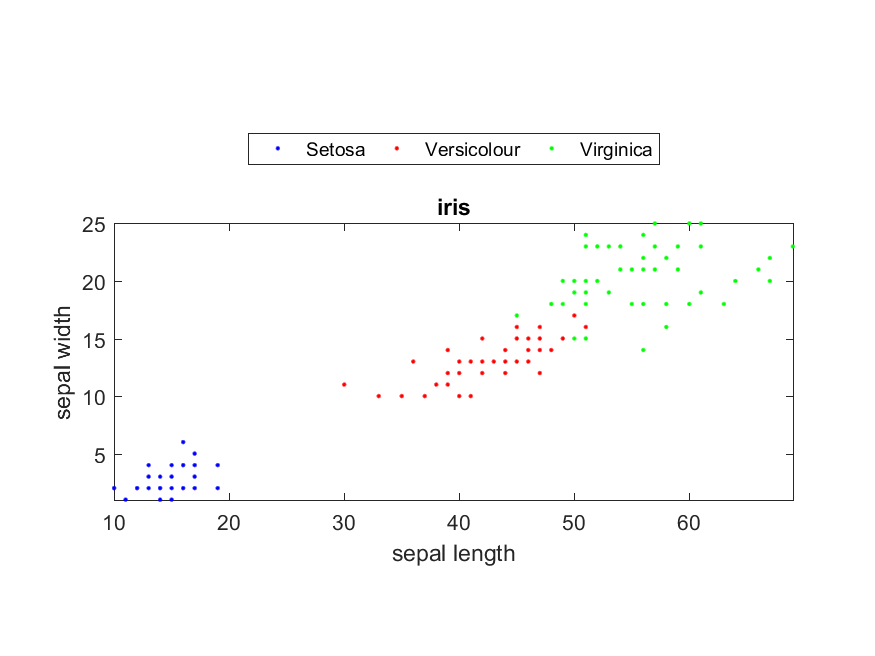In fact, the area of sepals and petals is an effective feature for classifying three species of iris. We can use the multiplication of sepal's width and length as the area of the sepal. And similar for petal. We can then use these area-based features to have the scatter plot, as follows:

Example 7: irisPlot2dfeaCombine.mds=prData('iris'); ds.input=[ds.input(1,:).*ds.input(2,:); ds.input(3,:).*ds.input(4,:)]; ds.inputName=''; ds.inputName{1}='Area of sepal'; ds.inputName{2}='Area of petal'; dsScatterPlot(ds);From the plot, it is obvious that Setosa is quite separated from the other two classes, while the other two classes have partial overlap at their boundaries.

We can have another scatter plot after projecting the dataset onto a 3D space:

Example 8: irisProjPlot3.mds = prData('iris'); dsProjPlot3(ds);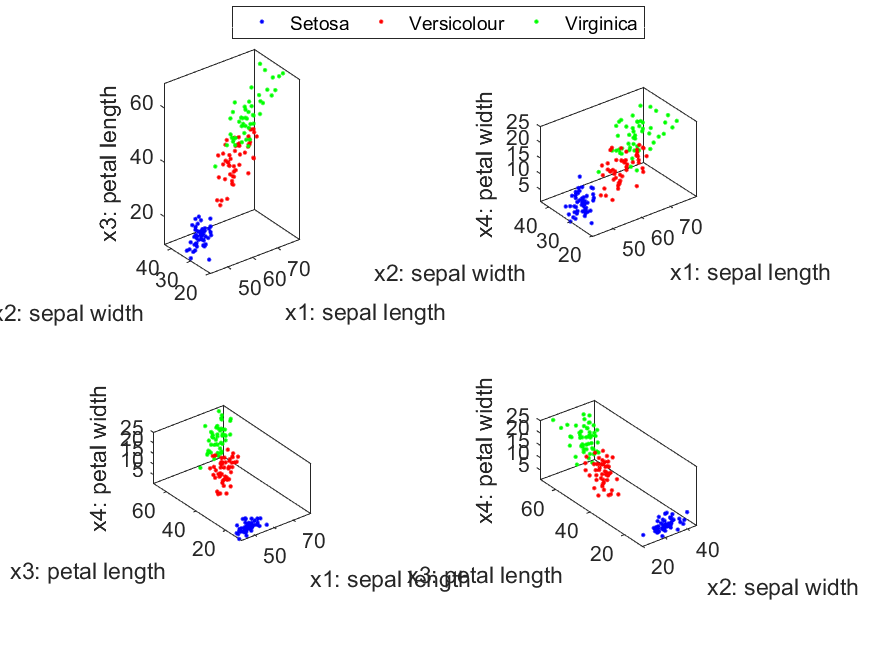Basically, we can only visualize data points scattered in a 2D plane or a 3D space. If we want to visualize data points in a 4D space, then the fourth feature can be viewed as the time and the other three features becomes moving points in a 3D space.

Data Clustering and Pattern Recognition (資料分群與樣式辨認)